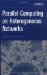6.10. A SIMPLE HETEROGENEOUS ALGORITHM SOLVING A REGULAR PROBLEM

6.9. THE RECON STATEMENT: A LANGUAGE CONSTRUCT TO CONTROL THE ACCURACY OF THE UNDERLYING MODEL OF COMPUTER NETWORK

The mapping of abstract processors of an mpC network to parallel processes of the program is based on the information about the speed at which the processes run on the physical processors of the executing network. By default, the mpC programming system uses the same estimation of speeds of the physical processors that was obtained earlier by the execution of a special parallel test program during the initialization of the mpC system on the network of computers.

In Chapter 5 of this book we mentioned that the estimation is not sufficiently accurate in parallel computing on common networks of computers because of the processors’ heterogeneity and their multiple users. An estimate may be far off from the actual speeds of processors during program execution if

• the executed code essentially differs from the code of this special test program, or

• the workload of computers substantially differs from their workload during the execution of the special parallel test program.

The mpC language provides the means for programmers to change at runtime the default estimation of the processor speed by tuning it to the computations that will actually be executed. The next program demonstrates this effect:

#include <stdio.h> #include <stdlib.h> #include <math.h> #include <mpc.h> #define DELTA (0.5) typedef struct    {       double len;       double wid;       double hei;       double mass;    } rail; nettype HeteroNet(int n, double v[n]) {    coord I=n;    node { I>=0: v[I]; };    parent ; }; double Density(double x, double y, double z) {    return 6.0*sqrt(exp(sin(sqrt(x*y*z)))); } double RailMass(double len, double wid, double hei,  double delta) {    double mass, x, y, z;    for(mass=0., x=0.; x<len; x+=delta)       for(y=0.; y<wid; y+=delta)         for(z=0.; z<hei; z+=delta)           mass+=Density(x,y,z);    return mass*delta*delta*delta; } int [*]main(int [host]argc, char **[host]argv) {    repl N=3;    if(argc>1)       N=[host]atoi(argv);    if(N<=0)    {       [host]printf("Wrong input (N=%d)\n", [host]N);       MPC_Exit(-1);    }    else {    static rail [host]steel_hedgehog[[host]N];    repl double volumes[N];    double [host]start;    int [host]i;    repl j;    for(i=0; i<[host]N; i++)    {       steel_hedgehog[i].len = 200.0*(i+1);       steel_hedgehog[i].wid = 5.0*(i+1);       steel_hedgehog[i].hei = 10.0*(i+1);    }    start = [host]MPC_Wtime();    for(j=0; j<N; j++)       volumes[j] = steel_hedgehog[j].len *             steel_hedgehog[j].wid *             steel_hedgehog[j].hei;    recon RailMass(20., 4., 5., 0.5);    {       net HeteroNet(N,volumes) mynet;       rail [mynet]myrail;       double [mynet]x, [mynet]y, [mynet]z;       myrail = steel_hedgehog[];       myrail.mass = [mynet] RailMass(myrail.len,                      myrail.wid,             myrail.hei, DELTA);    [mynet]MPC_Printf("Rail #%d is %gcm x %gcm x%gcm "             "and weights %g kg\n",             I coordof mynet, myrail.len,                      myrail.wid,myrail.hei, myrail.mass/ 1000.0);    [host]printf("The steel hedgehog weights %g kg\n",       [host]((myrail.mass)[+])/1000.0);    }    [host]printf("\nIt took %.1f seconds to run the program.\n",          [host]MPC_Wtime()-start); }

The program differs from the first program in Section 6.8 mainly by its introduction of a statement recon, which is executed just before the definition of the network mynet. All physical processors running the program are thus instructed to execute in parallel a piece of code provided by the statement, and the time elapsed by each of the processors to execute the piece of code is used to refresh the estimation of its speed.

In our case the piece of code is a call to function RailMass with arguments 20.0, 4.0, 5.0, and 0.5. The main part of the volume of computations performed by each abstract processor of network mynet falls exactly into the execution of calls to function RailMass. Therefore, while mapping abstract processors of network mynet, the mpC programming system will use such an estimation of speeds of physical processors that will be very close to their actual performance during the execution of this program.

It is important that the recon statement allow the programmer to refresh the estimation of processor speeds dynamically, at runtime, just before this estimation is used, and in particular, if the network of computers that executes the mpC program is used for other compuatations as well. The real speed of processors can dynamically change depending on their load by other computations external to the mpC program. Use of the recon statement allows the programmer to write parallel programs that are sensitive to such dynamic variation of the workload of the executing network. In such programs the computations are distributed over the physical processors in accordance with their actual computational performances.Parallel Computing on Heterogeneous Networks (Wiley Series on Parallel and Distributed Computing)
ISBN: B000W7ZQBO
EAN: N/A
Year: 2005
Pages: 95